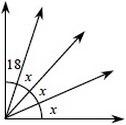### Home > MC1 > Chapter 10 > Lesson 10.1.5 > Problem10-62

10-62.

Draw two different diagrams that could be represented by the equation $3x +18 = 90$. Then find the value of $x$.

Below is one diagram to illustrate this equation. Can you draw another?$x = 24$